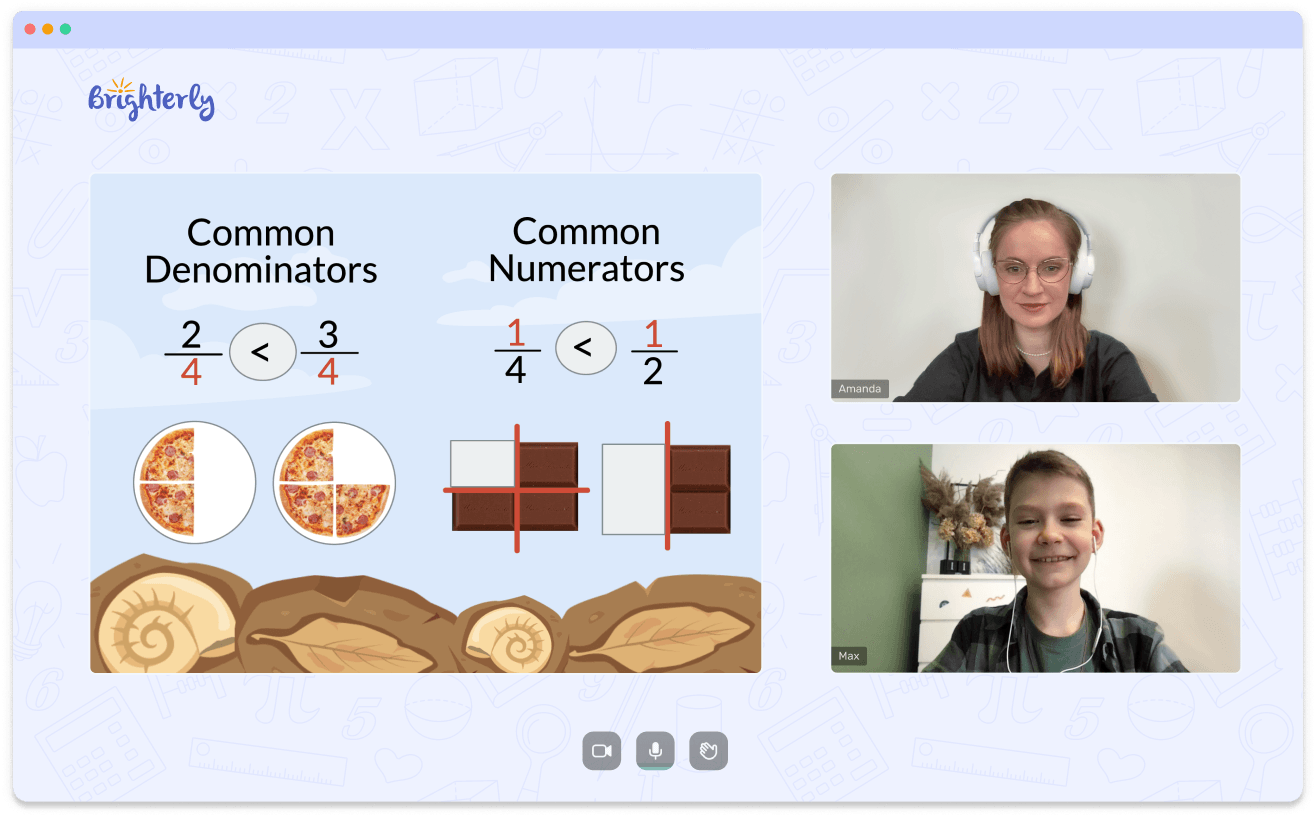# Percent Of A Number Worksheets

Your child’s comprehension of when and where to apply formulas to find percentages and utilize them to solve real-world situations is improved if you use worksheets.

Leave your email and we will send you worksheets

worksheets sent successfully

A percentage is a figure or ratio stated as a fraction of 100. The numerator and denominator of a fraction are the numbers of parts you have relative to the total number of parts. There is no way to measure the proportion since it is a number without dimensions.

A percent of a number worksheet allows pupils to practice computing percentages by multiplying the ratio’s numerical value by 100.

## Benefits of Percent of a Number Worksheets

The idea of percent will be introduced to your child through percent of a number worksheets. They will then discover how to contrast and arrange percent values. Finally, they will study formulas for percent equations, percent increase, and percent decrease issues.

Math for Kids

Is Your Child Struggling With Math?
1:1 Online Math TutoringFinding the percent of a number worksheet problems involve the calculation of simple interest, discounts, and markups, which are the tasks of advanced levels of this concept.

Practice with the worksheets promotes self-learning as well as time management and accuracy.

Your child will be learning independently by completing the finding a percent of a number worksheet with answers.

Each math learner needs to be proficient in accuracy and speed. To speed up and make wiser choices when answering arithmetic problems, your child needs to practice with a lot of finding percent of a number worksheet questions.

Leave your email and we will send you worksheets

worksheets sent successfully### Percent Of A Number Worksheets PDF

Percent Of A Number Worksheet### Percent Of A Number Worksheets PDF

Finding Percent Of A Number Worksheet### Percent Of A Number Worksheets PDF

Finding The Percent Of A Number Worksheet### Percent Of A Number Worksheets PDF

Percentage Of Number Worksheet

Using these worksheets, they acquire effective time management skills and can pinpoint the areas in which they need more practice or conceptual knowledge.

Because worksheets are a polished platform for learning, revision, and progress, a percentage of number worksheet is a crucial component of the learning process.

### More Percentages Worksheets

Book 1 to 1 Demo Lesson• We help children understand Math concepts instead of memorizing.
• We will adapt to your child needs to mprove math performance.

Is Your Child Struggling With Math?
Book 1 to 1 Demo Lesson